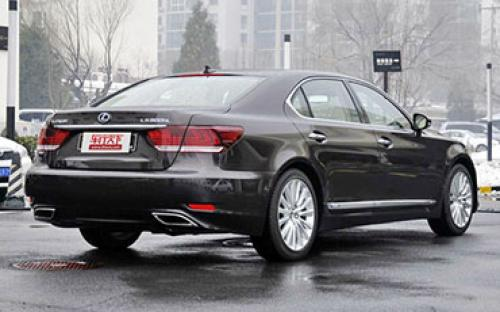### 雷克萨斯(进口) 雷克萨斯LS10 种颜色可选2014款最低售价：149.00 万元起

5210(mm)1875(mm)1465(mm)##### 配置亮点：
• 胎压监测装置

• ISOFIX儿童座椅接口

• 车身稳定控制(ESC/ESP/DSC等)

• 电动天窗

• 定速巡航

• 后倒车雷达

• 真皮座椅

• GPS导航系统

• 氙气大灯

• 后视镜加热

• 提交

雷克萨斯(进口) 雷克萨斯LS 绕车实拍• 雷克萨斯(进口) 雷克萨斯LS 在售车型

排量 车型 厂商指导价 本地最低报价 购车工具
4.6L
460L 8挡手自一体
149.00万
149.00万

460L 豪华版 8挡手自一体
163.80万
163.80万

460L 四驱豪华版 8挡手自一体
180.30万
180.30万

600hL 油电混合 CVT无级变速
238.80万
238.80万

5.0L
460L 8挡手自一体
149.00万
149.00万

460L 豪华版 8挡手自一体
163.80万
163.80万

460L 四驱豪华版 8挡手自一体
180.30万
180.30万

600hL 油电混合 CVT无级变速
238.80万
238.80万

雷克萨斯(进口) 雷克萨斯LS 经销商

查看更多 >>

### 雷克萨斯(进口) 雷克萨斯LS 动力加速

雷克萨斯LS 0-100公里加速时间分布在 6.1-6.5秒 属于 高性能级

动力级别 加速时间 车型
高性能级(3款)
6.1s
460L460L 豪华版
6.5s
460L 四驱豪华版
6.4s
600hL

雷克萨斯(进口) 雷克萨斯LS 视频

雷克萨斯(进口) 雷克萨斯LS 新闻资讯

# 雷克萨斯发布新款LS系列官图 配置升级

新闻 超过4837次关注

日前，雷克萨斯发布了新款LS的官图和新车信息，这款车的配置相比现款有所升级。

# 雷克萨斯召回部分LS/GS/IS 共计1880辆

新闻 超过4978次关注

日前，丰田汽车（中国）投资有限公司向国家质检总局备案了召回计划，自2014年11月14日起，召回部分进口雷克萨斯（LEXUS）LS600h/GS450h/IS250/IS250C车型。

# 车展体验雷克萨斯LS600hL 新旗舰车型

车系文章 超过3509次关注

在11月22日开幕的广州车展上，LS中的旗舰——LS600hL正式在国内发布，并将于明年正式引进。新车外观内饰做了重新设计，并且采用很多高科技配置，可谓变化巨大。

# 实拍雷克萨斯全新LS600hL 豪华大气又环保

车系文章 超过3438次关注

作为雷克萨斯的旗舰车型，雷克萨斯LS600h在2006年的纽约车展就已经公开亮相，在豪华车市场刮起一股环保风。值得一提的是，雷克萨斯LS600hL不仅是世界上首辆搭载V8发...

# 舒适与环保的结合体 测雷克萨斯LS600hL

车系文章 超过3520次关注

在国内的豪华车市场上，我们一直将注意力更多的放在传统德系三强身上。从奥迪A8的换代、全新奔驰S级的上市，再到宝马7系近期频繁曝出的谍照。这三家老牌厂商动作频...

# 雷克萨斯LS Sports 650将2015年量产

车系文章 超过2148次关注

日前，据海外媒体报道，雷克萨斯LSSports650概念车将于2015年量产，这款车是由丰田欧洲赛事部门TMG打造的，未来的竞争对手锁定为奥迪S8以及奔驰S63AMG车型。

# 雷克萨斯LS新车上市 52.70-238.8万元

车系文章 超过2375次关注

日前，雷克萨斯官方宣布旗下包含新款GX400、2014款LS系列（LS460L、LS600hL）以及2014款GS系列（GS250、GS350、GS450h）车型正式上市，一共包括11款车型。新款GX400...

# 解读LED大灯技术 优点在哪里

车系文章 超过2174次关注

都说眼睛是心灵的窗户，对于汽车而言，有一对漂亮的大灯，就像拥有一双漂亮的眸子一样摄人心扉。LED大灯的出现就像是给车头灯配上了“美瞳”，把“眼睛”的魅力指数...

猜你喜欢

﻿
• 快速找车
• 选择品牌
• 选择品牌
• A  奥迪
• A  阿斯顿·马丁
• A  阿尔法·罗密欧
• B  宝沃
• B  布加迪
• B  巴博斯
• B  保时捷
• B  宾利
• B  奔驰
• B  宝马
• B  本田
• B  别克
• B  标致
• B  比亚迪
• B  宝骏
• B  北汽制造
• B  北汽新能源
• B  北汽幻速
• B  北汽威旺
• B  北京汽车
• B  奔腾
• B  北汽绅宝
• C  长安
• C  长安商用
• C  长城
• C  昌河
• D  大众
• D  道奇
• D  DS
• D  东南
• D  东风风神
• D  东风风行
• D  东风小康
• D  东风风度
• D  东风
• F  福特
• F  丰田
• F  菲亚特
• F  法拉利
• F  福田
• F  福迪
• F  福汽启腾
• G  观致
• G  广汽传祺
• G  广汽吉奥
• G  GMC
• H  红旗
• H  汉腾汽车
• H  哈弗
• H  哈飞
• H  海格
• H  海马
• H  华颂
• H  黄海
• H  华泰
• H  恒天
• J  吉利汽车
• J  捷豹
• J  Jeep
• J  江淮
• J  江铃
• J  金杯
• J  九龙
• J  金旅
• K  凯翼
• K  凯迪拉克
• K  克莱斯勒
• K  科尼塞克
• K  卡威
• K  开瑞
• L  路虎
• L  林肯
• L  劳斯莱斯
• L  兰博基尼
• L  雷克萨斯
• L  铃木
• L  雷诺
• L  理念
• L  力帆
• L  莲花汽车
• L  猎豹
• L  路特斯
• L  陆风
• M  马自达
• M  MG
• M  MINI
• M  玛莎拉蒂
• M  摩根
• M  迈凯轮
• N  纳智捷
• O  欧宝
• O  讴歌
• O  欧朗
• Q  奇瑞
• Q  起亚
• Q  启辰
• R  日产
• R  荣威
• R  瑞麒
• S  三菱
• S  斯威汽车
• S  萨博
• S  smart
• S  斯柯达
• S  斯巴鲁
• S  思铭
• S  双龙
• S  上汽大通
• S  双环
• T  特斯拉
• T  腾势
• W  沃尔沃
• W  五菱汽车
• W  五十铃
• W  威兹曼
• W  威麟
• X  现代
• X  雪佛兰
• X  雪铁龙
• X  西雅特
• Y  一汽
• Y  英菲尼迪
• Y  英致
• Y  依维柯
• Y  野马汽车
• Y  永源
• Z  众泰
• Z  中华
• Z  中兴
• Z  知豆
• 选择车系
• 选择车系
• 车型对比
• 选择品牌
• 选择品牌
• A  奥迪
• A  阿斯顿·马丁
• A  阿尔法·罗密欧
• B  宝沃
• B  布加迪
• B  巴博斯
• B  保时捷
• B  宾利
• B  奔驰
• B  宝马
• B  本田
• B  别克
• B  标致
• B  比亚迪
• B  宝骏
• B  北汽制造
• B  北汽新能源
• B  北汽幻速
• B  北汽威旺
• B  北京汽车
• B  奔腾
• B  北汽绅宝
• C  长安
• C  长安商用
• C  长城
• C  昌河
• D  大众
• D  道奇
• D  DS
• D  东南
• D  东风风神
• D  东风风行
• D  东风小康
• D  东风风度
• D  东风
• F  福特
• F  丰田
• F  菲亚特
• F  法拉利
• F  福田
• F  福迪
• F  福汽启腾
• G  观致
• G  广汽传祺
• G  广汽吉奥
• G  GMC
• H  红旗
• H  汉腾汽车
• H  哈弗
• H  哈飞
• H  海格
• H  海马
• H  华颂
• H  黄海
• H  华泰
• H  恒天
• J  吉利汽车
• J  捷豹
• J  Jeep
• J  江淮
• J  江铃
• J  金杯
• J  九龙
• J  金旅
• K  凯翼
• K  凯迪拉克
• K  克莱斯勒
• K  科尼塞克
• K  卡威
• K  开瑞
• L  路虎
• L  林肯
• L  劳斯莱斯
• L  兰博基尼
• L  雷克萨斯
• L  铃木
• L  雷诺
• L  理念
• L  力帆
• L  莲花汽车
• L  猎豹
• L  路特斯
• L  陆风
• M  马自达
• M  MG
• M  MINI
• M  玛莎拉蒂
• M  摩根
• M  迈凯轮
• N  纳智捷
• O  欧宝
• O  讴歌
• O  欧朗
• Q  奇瑞
• Q  起亚
• Q  启辰
• R  日产
• R  荣威
• R  瑞麒
• S  三菱
• S  斯威汽车
• S  萨博
• S  smart
• S  斯柯达
• S  斯巴鲁
• S  思铭
• S  双龙
• S  上汽大通
• S  双环
• T  特斯拉
• T  腾势
• W  沃尔沃
• W  五菱汽车
• W  五十铃
• W  威兹曼
• W  威麟
• X  现代
• X  雪佛兰
• X  雪铁龙
• X  西雅特
• Y  一汽
• Y  英菲尼迪
• Y  英致
• Y  依维柯
• Y  野马汽车
• Y  永源
• Z  众泰
• Z  中华
• Z  中兴
• Z  知豆
• 选择车系
• 选择车系
• 选择车型
• 选择车型
• 意见反馈